# Class 12 Maths NCERT Solutions for Chapter 10 Vector Algebra Exercise 10.3### Vector Algebra Exercise 10.3 Solutions

1. Find the angle between two vectors a⃗ and b⃗ with magnitudes √3 and 2, respectively having a⃗ .  b⃗ = √6.

Solution

It is given that,
| a⃗ | = √3, | b⃗ | = 2 and,  a⃗ . b⃗  = √6 .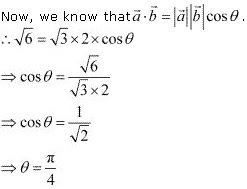Hence, the angle between the given vectors  a⃗  and  b⃗ is π/4.

2. Find the angle between the vectors  i - 2j ^  + 3k^  and  3i - 2j ^  + k^   .

Solution

The given vectors are  a = i - 2j ^  + 3k^  and  b = 3i - 2j ^  + k^  .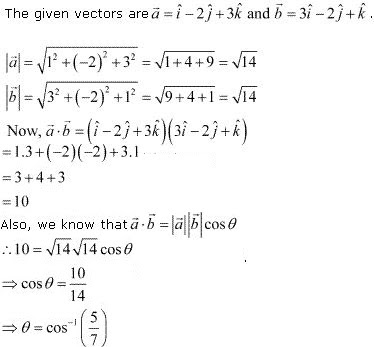3. Find the projection of the vector  i - j ^  on the vector  i + j ^.

Solution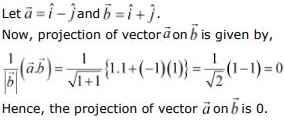4. Find the projection of the vector  i + 3j ^  + 7k^  on the vector 7i - j ^ + 8k^ .
Solution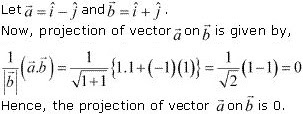5. Show that each of the given three vectors is a unit vector :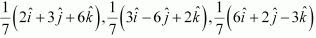Also, show that they are mutually perpendicular to each other.
Solution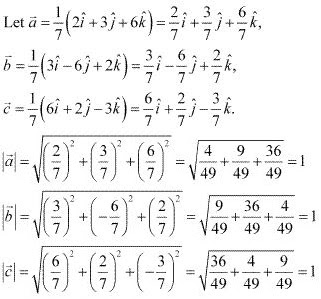Thus, each of the given three vectors is a unit vector.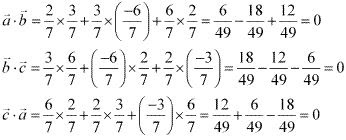Hence, the given three vectors are mutually perpendicular to each other.

6. Find | a⃗ | and | b⃗ |,  if ( a⃗ +  b) . ( a⃗ - b⃗ ) = 8 and  |a⃗ | = 8| b⃗ |.
Solution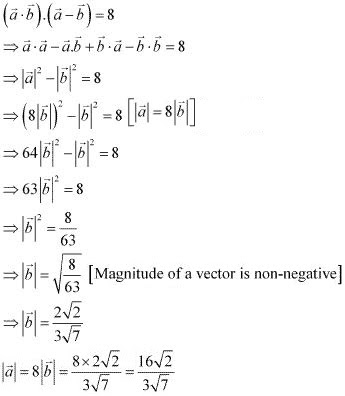7. Evaluate the product  ( 3a⃗ - 5b⃗ ) . ( 2a⃗ +  7b⃗ ).
Solution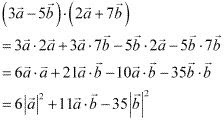8. Find the magnitude of two vectors a⃗ and  b⃗ , having the same magnitude and such that the angle between them is 60° and their scalar product is  1/2 .
Solution
Let θ be the angle between the vectors    a⃗ and  b⃗
It is given that  | a⃗ | = | b⃗ |,  a⃗ .  b⃗   = 1/2 , and θ = 60° ...(1)
We know that  a⃗ . b⃗  = | a⃗ | | b⃗ | cos θ.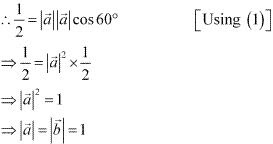9. Find | x⃗ |, if for a unit vector  a⃗ , ( x⃗ -  a⃗ ).( x⃗ +  a⃗ ) = 12.
Solution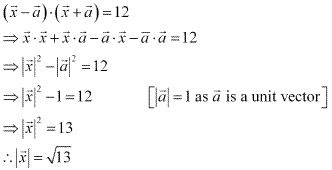10. If  a = 2i - 2j ^  + 3k^ ,  b =  - i + 2j ^ + k^  and  c = 3i + j ^ are such that  a⃗  = λb⃗ is perpendicular c⃗ , then find the value of  λ .
Solution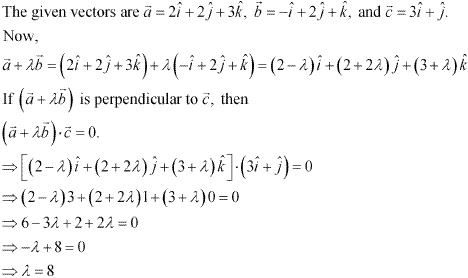Hence, the required value of λ is 8.

11. Show that (|a⃗ |b⃗  ) + (|b⃗ |a )is perpendicular to (|a⃗ |b⃗ ) - (|b⃗ |a), for any two nonzero vectors    a⃗ and  b.
Solution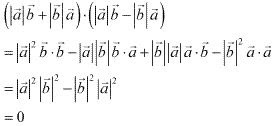Hence, | a⃗ |b + | b⃗ |a and | a⃗ |b⃗ - | b⃗ |a⃗  are perpendicular to each other.

12. If  a⃗ . a⃗ = 0 and  a⃗ . b⃗ = 0, then what can be concluded about the vector b⃗
Solution
It is given that a. a⃗ = 0 and  a⃗ . b⃗ = 0
Now,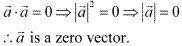Hence, vector b⃗ satisfying a⃗ . b⃗ = 0 can be any vector.

13. If  a⃗ , b⃗  and  c⃗  are unit vectors such that  a⃗ + b⃗  + c =  0⃗ , find the value of  a⃗ . b⃗ +  b⃗ . c⃗ +  c⃗ .  a⃗ .
Solution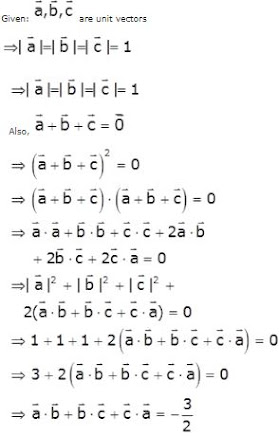14. If either vector a⃗ = 0⃗ or b⃗ =  0 then a⃗ ,  b = 0. But the converse need not be true. justify your answer with an example.
SolutionHence, the converse of the given statement need not be true.

15. If the vertices A, B, C of a triangle ABC are (1, 2, 3), (–1, 0, 0), (0, 1, 2), respectively, then find ∠ABC. [∠ABC is the angle between the vectors and BA⃗ and BC⃗
Solution

The vertices of ΔABC are given as A (1, 2, 3), B (–1, 0, 0), and C (0, 1, 2).
Also, it is given that ∠ABC is the angle between the vectors   BA⃗ and  BC⃗   .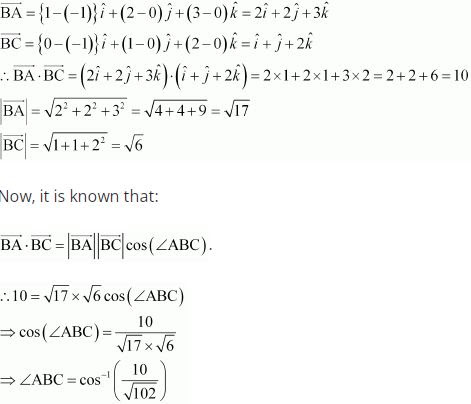16. Show that the points A (1, 2, 7), B (2, 6, 3) and C (3, 10, –1) are collinear.

Solution

The given point are A (1, 2, 7), B (2, 6, 3) and C (3, 10, –1) .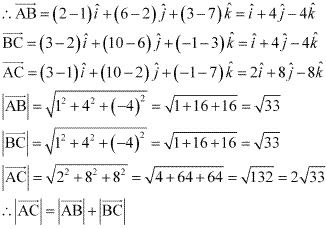Hence, the given points A, B, and C are collinear.

17. Show that the vectors 2i ^- j ^+k^ , i ^-3j ^-5k^  and 3i ^- 4j ^-4k^ from the vertices of a right angled triangle.

Solution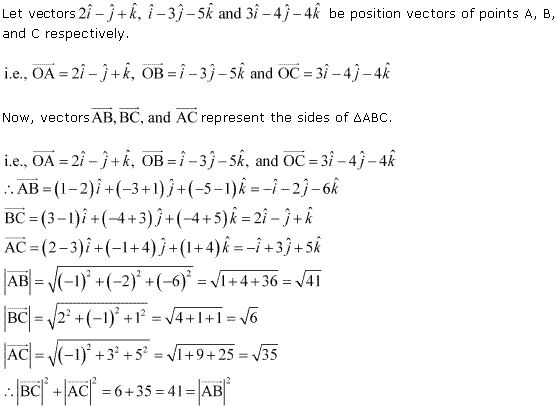Hence, Δ ABC is a right angled triangle.

18. If  a⃗  is a nonzero vector of magnitude 'a' and λa⃗  is unit vector if
(A) λ = 1
(B) λ = -1
(C) a = |λ|
(D) a = 1/|λ|
Solution
Vector λa⃗  is a unit vector if |λa| = 1 .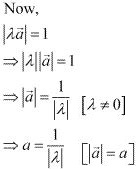Hence, vector λa⃗ is a unit vector if a = 1/|λ|.